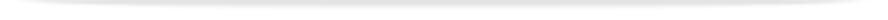units

ENG1005

Faculty of Engineeringprint version

This unit entry is for students who completed this unit in 2016 only. For students planning to study the unit, please refer to the unit indexes in the the current edition of the Handbook. If you have any queries contact the managing faculty for your course or area of study.

ENG1005 - Engineering mathematics

6 points, SCA Band 2, 0.125 EFTSL

Refer to the specific census and withdrawal dates for the semester(s) in which this unit is offered.

Faculty

Engineering

Coordinator(s)

Dr Leo Brewin and Dr John Head (Clayton - Sem One); Dr John Head (Clayton - Sem Two); Associate Professor Lan Boon Leong (Malaysia)

Offered

Clayton

• First semester 2016 (Day)
• Second semester 2016 (Day)

Malaysia

• First semester 2016 (Day)
• Second semester 2016 (Day)
• October intake 2016 (Day)

Synopsis

Vector algebra and geometry: equations of lines and planes. Linear algebra: matrix operations, up to 3x3 systems of linear equations, eigenvalues and eigenvectors. Calculus: improper integrals, integration by parts. Sequences and series: fundamentals of convergence, Taylor series, use in error analysis. Ordinary differential equations: first order, second order with constant coefficients, repeated roots, simple non-homogeneous cases. Laplace transforms: elementary functions, inversion by tables; shifting; derivatives, applications to ODEs. Multivariable calculus: partial derivatives, gradient and directional derivatives, maxima and minima.

Outcomes

On successful completion of this unit, students will be able to:

1. Evaluate cross products of vectors, and use vectors to represent lines and planes.
2. Perform matrix algebra.
3. Solve up to 3x3 systems of linear equations and find eigenvalues and eigenvectors.
4. Use hyperbolic functions.
5. Evaluate improper integrals of elementary functions and use integration by parts.
6. Appreciate convergence of numeric and power series, construct Taylor series and estimate errors in numerical approximations .
7. Solve first order ordinary differential equations, including by separable variables and integrating factors.
8. Solve second order linear differential equations with constant coefficients.
9. Use differential equations to model simple engineering problems.
10. Evaluate and invert Laplace transforms and use them to solve ordinary differential equations.
11. Calculate partial derivatives, use the gradient vector to find directional derivatives, and find extreme values of two-variable functions.
12. Express and explain mathematical techniques and arguments clearly in words.

Assessment

Weekly assignments or quizzes: 30%
Final examination (3 hours): 70%

Students are required to achieve at least 45% in the total continuous assessment component and at least 45% in the final examination component and an overall mark of 50% to achieve a pass grade in the unit. Students failing to achieve this requirement will be given a maximum of 45% in the unit.

Three 1-hour lectures (or equivalent), one 2-hour practice class and 7 hours of private study per week

Chief examiner(s)

Dr Leo Brewin (Sem 1+2)
Dr Leo Brewin (Malaysia October intake 2016)

Prerequisites

VCE Specialist Mathematics or ENG1090 (or equivalent)

Prohibitions

ENG1091, MTH1030, MTH1035